# Calculus 1 : Other Differential Functions

## Example Questions

### Example Question #91 : How To Find Differential Functions

Differentiate the function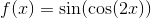None of these answers are correct.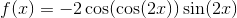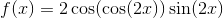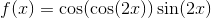Explanation:

To differentiate the function properly, we must use the Chain Rule which is,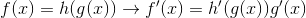Therefore the derivative of the function is,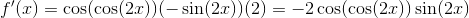### Example Question #91 : Other Differential Functions

Differentiate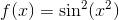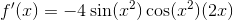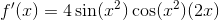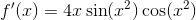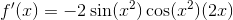Explanation:

To differentiate this equation we use the Chain Rule.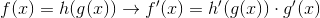Using this throughout the equation gives us,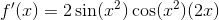### Example Question #93 : Other Differential Functions

Find the derivative of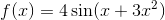None of these answers are correct.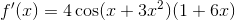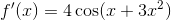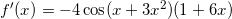Explanation:

To find the derivative of the function we must use the Chain Rule, which isApplying this to the function we get,### Example Question #91 : How To Find Differential Functions

Find the derivative of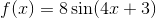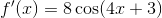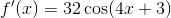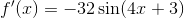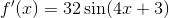Explanation:

To find the derivative of the function we must use the Chain RuleApplying this to the function we are given gives,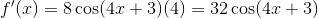### Example Question #92 : Other Differential Functions

Find the first derivative of the function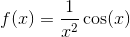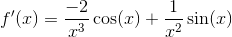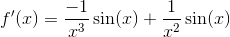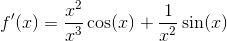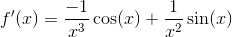Explanation:

To find the derivative of this function we can use the Product Rule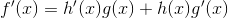Applying this to the function we get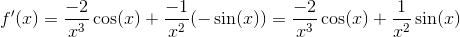### Example Question #96 : Other Differential Functions

Differentiate the following function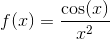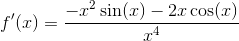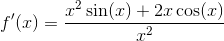None of these answers are correct.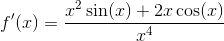Explanation:

To differentiate the function that we are given we must use the Quotient Rule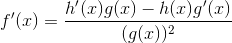Applying this to the function we are given gives,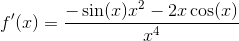### Example Question #93 : Other Differential Functions

Find the derivative of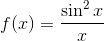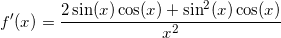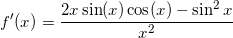None of these answers are correct.Explanation:

To differentiate this function we must use the Chain Rule and the Quotient RuleApplying these to the function we are given gives us,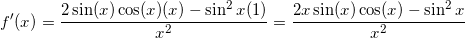### Example Question #94 : Other Differential Functions

Find the first derivative of the function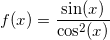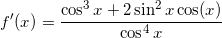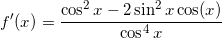None of these answers are correct.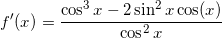Explanation:

To differentiate this function we must use the Quotient Rule whereUsing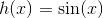and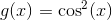with the Quotient Rule gives,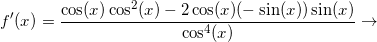### Example Question #95 : Other Differential Functions

Differentiate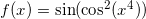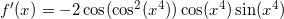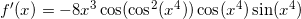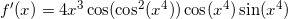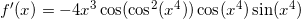Explanation:

To differentiate this function we must use the Chain Rule.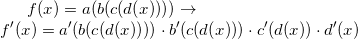Applying this to the function we obtain,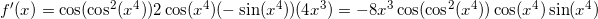### Example Question #96 : Other Differential Functions

Differentiate the polynomial.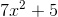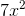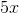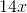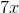Using the power rule, we can differentiate our first term reducing the power by one and multiplying our term by the original power., will thus become. The second term is a constant value, so according to the power rule this term will become.